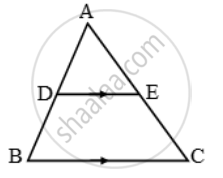# In the given figure, DE || BC and DE : BC = 3 : 5. Calculate the ratio of the areas of ∆ADE and the trapezium BCED - Mathematics

Sum

In the given figure, DE || BC and DE : BC = 3 : 5. Calculate the ratio of the areas of ∆ADE and the trapezium BCED#### Solution

\therefore \frac{ar(\Delta ADE)}{ar(\Delta ABC)}=(DE^2)/(BC^2)=((DE)/(BC))^2=(3/5)^2=9/25

Let ar (∆ADE) = 9x sq units

Then, ar (∆ABC) = 25x sq units

ar (trap. BCED) = ar (∆ABC) – ar (∆ADE)

= (25x – 9x) = (16x) sq units

\therefore \frac{ar(\Delta ADE)}{ar(trap.BCED)}=\frac{9x}{16x}=\frac{9}{16}

Concept: Areas of Similar Triangles
Is there an error in this question or solution?
Chapter 7: Triangles - Exercise 7.6 [Page 95]

#### APPEARS IN

RD Sharma Class 10 Maths
Chapter 7 Triangles
Exercise 7.6 | Q 8.3 | Page 95

Share